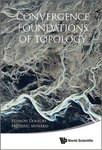Mathematical Sciences Faculty BookshelfTitle

Convergence Foundations of Topology

Description

Georgia Southern University faculty member Frédéric D. Mynard co-authored Convergence Foundations of Topology.

Book Summary: The textbook is an alternative to a classical introductory book in point-set topology. The approach, however, is radically different from the classical one. It is based on convergence rather than on open and closed sets. On the one hand, convergence of filters is a natural generalization of the basic and well-known concept of convergence of sequences, so that convergence theory is more natural and intuitive to many, perhaps most, students than classical topology. On the other hand, the framework of convergence is easier, more powerful and far-reaching which highlights a need for a theory of convergence in various branches of analysis. Convergence theory for filters is gradually introduced and systematically developed. Topology spaces are introduced as a special subclass of convergence spaces of particular interest, but a large part of the material usually developed in a topology textbook is treated in the larger realm of convergence spaces. A number of exercises (with solutions) form an essential part of the text. Additional problems for each chapter are included (without solutions).

1-1-2009

Publisher

World Scientific Publishers

ISBN for this edition (13-digit)

9789814571524COinS